Diary — July 2021

July 1, 2021I must be in Berkeley. I heard a horrible scream out back as they slaughtered a carrot.

July 8, 2021

Here's a good challenge: understanding $$\mathrm{Set}^{\mathrm{op}}$$, the opposite of the category of sets.

$$\mathrm{Set}^{\mathrm{op}}$$ has sets as objects but 'cofunctions' as morphisms. A cofunction $$f\colon X \rightarrow Y$$ is a function from Y to X. You compose cofunctions just like functions, only backwards.

Cool fact: $$\mathrm{Set}^{\mathrm{op}}$$ is equivalent to a certain category of very nice boolean algebras. A boolean algebra is a set with operations 'and', 'or', and 'not' obeying all the usual laws of classical logic.

Do you know how any set gives a boolean algebra?

Here's how: any set $$X$$ gives a boolean algebra $$PX$$, the power set of $$X$$, whose elements are subsets of $$X$$. In this boolean algebra 'and' is intersection, 'or' is union and 'not' is complement.

Turning sets into boolean algebras exposes how the category $$\mathrm{Set}$$ is all about logic.

Even better, any function $$f\colon X \rightarrow Y$$ gives a map $$Pf \colon PY \rightarrow PX$$ sending each subset of $$Y$$ to its inverse image under f.

Inverse image preserves intersection, union and complement, so $$Pf$$ is a 'boolean algebra homomorphism'.

Note how $$Pf$$ goes backwards from PY to $$PX$$. Given functions $$f \colon X \rightarrow Y$$ and $$g \colon Y \rightarrow Z$$ we have $$P(gf) = P(f) P(g)$$ so $$P$$ is a functor from $$\mathrm{Set}$$ to $$\mathrm{BoolAlg}^{\mathrm{op}}$$, the opposite of the category of boolean algebras. You could say boolean logic is set theory in reverse!

However, we don't get every boolean algebra this way, just very nice ones called 'complete atomic' boolean algebras. (Should I explain them?) These form a category $$\mathrm{CABA}$$, and our functor $$P \colon \mathrm{Set} \rightarrow \mathrm{BoolAlg}^{\mathrm{op}}$$ gives an equivalence which by abuse of language I'll call $$P \colon \mathrm{Set} \rightarrow \mathrm{CABA}^{\mathrm{op}}$$ Even if you don't know what a complete atomic boolean algebra is, this explains why we care. Our equivalence $$P \colon \mathrm{Set} \rightarrow \mathrm{CABA}^{\mathrm{op}}$$ can also be seen as an equivalence $$P \colon \mathrm{Set}^{\mathrm{op}} \rightarrow \mathrm{CABA}$$ So $$\mathrm{Set}^{\mathrm{op}}$$ is just $$\mathrm{CABA}$$ in disguise! This helps us understand $$\mathrm{Set}^{\mathrm{op}}$$.

Okay, okay... since you insist:

A boolean algebra is complete if we can take the 'or' not of just two elements, but of any collection of elements, no matter how large. $$PX$$ is complete because we can take the union of any collection of subsets of $$X$$.

We can define 'implies' in any boolean algebra, and 'false'. An atom is an element of a boolean algebra that's not false, and only implies itself and 'false'. The atoms in $$PX$$ are precisely the 1-element subsets of X. They're the smallest nonempty subsets.

A boolean algebra is atomic if every element equals the 'or' of all the atoms that imply it. $$PX$$ is atomic since every subset of $$X$$ is the union of all the 1-element sets that it contains.

So, any power set is a complete atomic boolean algebra! It's harder to show any complete atomic boolean algebra is isomorphic to a power set. But that's true too.

With more work we can define a category $$\mathrm{CABA}$$ of complete atomic boolean algebras, and show $$P \colon \mathrm{Set} \rightarrow \mathrm{CABA}^{\mathrm{op}}$$ is an equivalence, giving $$\mathrm{Set}^{\mathrm{op}} \simeq \mathrm{CABA}$$.

So, we can think of an object of $$\mathrm{Set}^{\mathrm{op}}$$ as a set with extra operations: a complete atomic boolean algebra. And we can think of a cofunction as a map that preserves these operations!

This is the start of a deeper story... which, alas, will not be told now.

July 11, 2021

Strontium is right below calcium in the periodic table, so it's chemically similar. If you eat chemicals containing strontium, it gets incorporated in your bones! This is very dangerous for radioactive strontium, which occurs in fallout from nuclear weapons. But for the four stable isotopes of strontium, it's okay to have a bit in your bones. For most of us, between 1 and 2 parts per thousand of the calcium in our bones has been replaced by strontium! But too much causes bone growth problems in kids.

The cool part: in the ocean there are lots of tiny organisms whose skeletons are made of strontium sulfate! Acantharea, they're the only known form of life that relies on strontium. They don't form fossils, since strontium sulfate dissolves too easily.

Another cool fact from Robin Houston: the word 'strontium' traces its origin to a Scottish phrase meaning "nose of the small hill that fairies live in". Read his tweets for more on this.

The picture of various organisms of genus Acantharea is from Ernst Haeckel's Kunstformen der Natur.

July 12, 2021

Jean Richafort (c.1480 – c.1547) is a Netherlandish composer in the third generation of the Franco-Flemish school, who studied with Josquin des Prez. The King's Singers have an album of his music called Jean Richafort: Requiem - Tributes to Josquin Desprez. It's very nice.

It starts with five pieces by Benedictus Appenzeller, Nicolas Gombert, Josquin des Prez, Jacquet of Mantua and Hieronymous Flinders, respectively. But then it gets down to business with the Requieum in memoriam Josquin des Prez, before concluding with Nymphes, nappés by Josquin.

The Huelgas Ensemble also has an album containing this requiem, called Requiem - In Memoriam Josquin Desprez. It should be fun to compare this performance:

July 15, 2021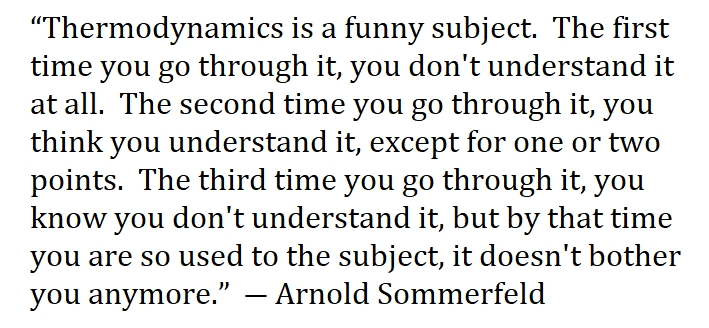This is a physicist's reaction to thermodynamics.

Most mathematicians quit after the first time.

July 16, 2021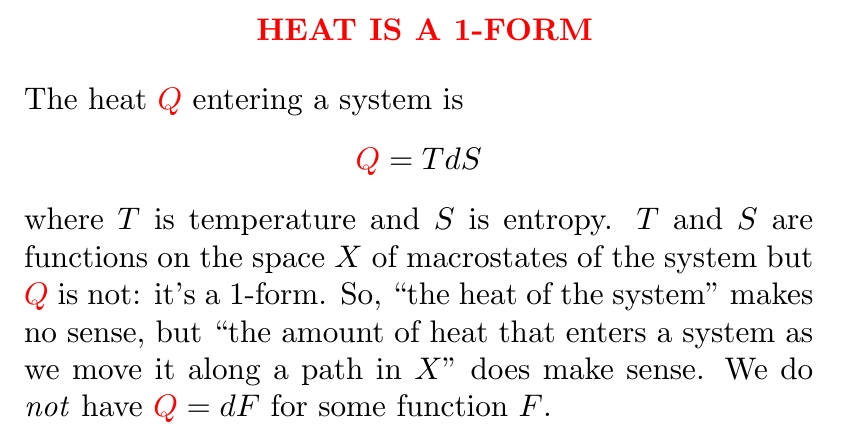A 1-form is something you integrate along a path: for example the "$$f(x) dx$$" you see inside an integral is a 1-form.

Heat is not a function: it's a 1-form. This confused everyone for a while, and it still confuses plenty of people now. Sometimes people call heat $$dQ$$, which is just wrong. Sometimes they call it $$dQ$$ with a little slash through the $$d$$, or $$\delta Q$$, which are better but still a bit mysterious, because they still suggest that there's some function $$Q$$. There's just a 1-form, which happens to equal $$T dS$$, and above I'm just calling that $$Q$$.

The connection between thermodynamics and differential forms turns out to be very important! For a bit more of an explanation, see the question here, and the first answer:

Here the questioner calls heat $$\delta Q$$, but this is the same as the thing I'm calling $$Q$$.

July 17, 2021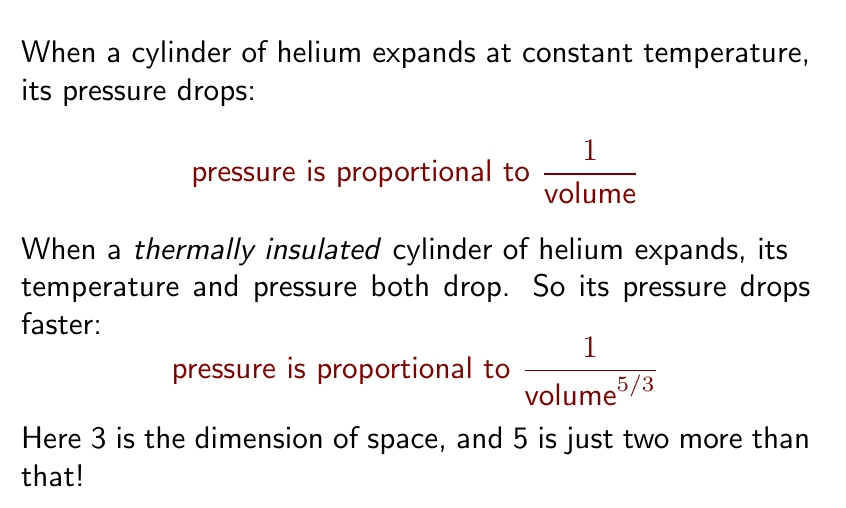The pressure of helium drops in funny way when you make it expand while preventing heat from flowing in. Pressure is inversely proportional to volume to the $$\frac{5}{3}$$ power.

Weird number! If space were 4-dimensional this would be $$\frac{6}{4}$$, and in 5 dimensions it would be $$\frac{7}{5}$$.

I explain why on my blog — I'm studying thermodynamics and explaining it to myself:

The key is that helium is a 'monatomic' gas, with just one atom per molecule, so in 3d space each molecule has energy $$\frac{3}{2}kT$$ where $$T$$ is the temperature and $$k$$ is Boltzmann's constant, a factor converting from temperature to energy.

In the number $$\frac{3}{2}$$, the $$3$$ is the dimension of space and the $$\frac{1}{2}$$ comes from the equipartition theorem: very roughly, if something can wiggle in $$n$$ directions its energy is $$\frac{n}{2}kT$$. Somehow this $$\frac{3}{2}$$ gives the $$\frac{5}{3}$$ in the final formula: read my blog for why.

But we don't need to go to other dimensions to see other rules for the expansion of an insulated cylinder of gas! For a diatomic gas like oxygen, each molecule can wiggle in $$5$$ directions so its energy is $$\frac{5}{2}kT$$. Its pressure is inversely proportional to its volume to the $$\frac{7}{5}$$.

Air is mainly made of diatomic gases: nitrogen and oxygen. So the pressure of an insulated cylinder of air is inversely proportional to its volume to the $$\frac{7}{5}$$... just like a monatomic gas in 5 dimensions!

It's fun to see how these peculiar numbers come from basic physics.

July 20, 2021

The Draa KsarWho built this in the desert of south-central Algeria, and why? Nobody knows. It's called a 'ksar', a fortified village — but it has just one entrance, and a double wall.What was worth defending so much? Was the climate moister then?

Some think the Draa ksar was a caravanserai — a place where caravans would stop and rest. Some would even have libraries. But this one looks different.

Apparently a team of Americans studied the Draa ksar for a week in the 1980s — but I don't who they were or what they found!The nearest town is Timimoun. It's actually on Tripadvisor, with six hotels. Maybe you could hire a camel driver and visit the ksar. But I think I'll sit this one out. It can be nice to imagine adventures you don't actually do.

The most I've been able to read about the Draa ksar is here:

July 21, 2021

Tai-Danae Bradley has a new paper that explains how entropy arises naturally from what I'll call the 'probability operad' — she calls it the operad of topological simplexes:
And she has a blog article that sketches some of the background, with pictures:

Since I love this stuff, I can't resist explaining a bit of it.

An operad has a bunch of $$n$$-ary operations for $$n = 0,1,2,3,\dots$$. You can plug the output of $$n$$-ary operation into any of the inputs of an $$m$$-ary operation and get an $$(n+m-1)$$-ary operation. The idea becomes clear in this picture shamelessly lifted from Bradley: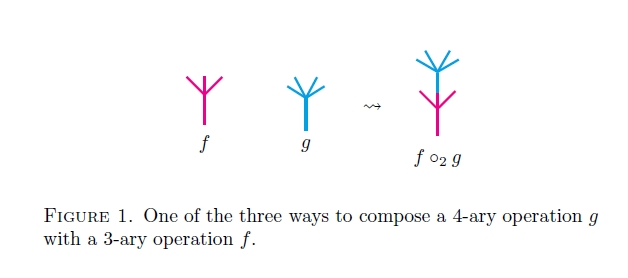The $$n$$-ary operations in the 'probability operad' are just probability distributions on the $$n$$-element set $$\{1,2,\dots, n\}$$. The cool part is how you plug one probability distribution into another. It's something we often do in real life!

Tai-Danae explains the idea here: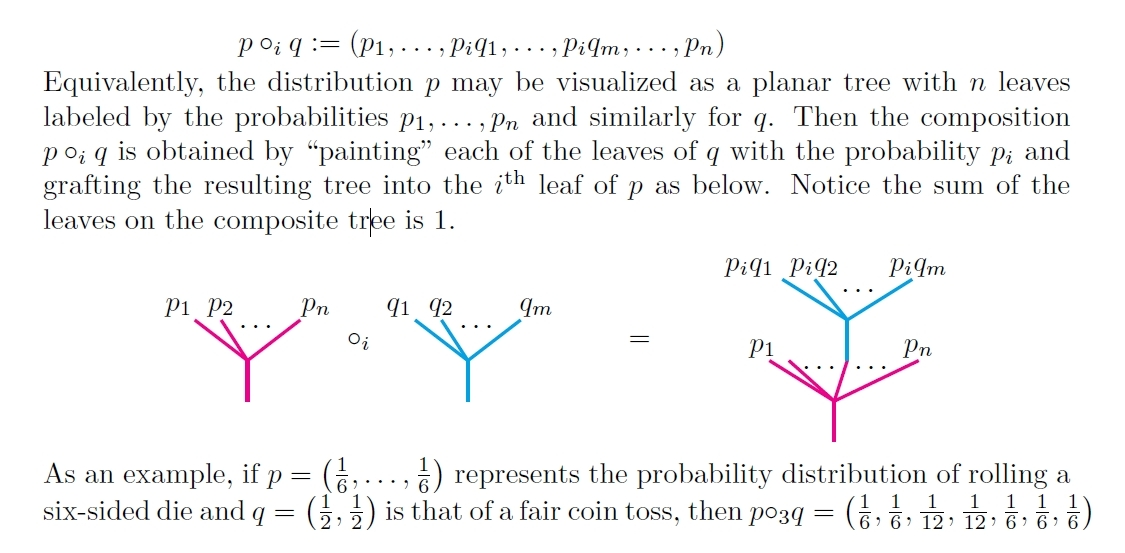For example, suppose I say "there's a 40% chance I'll eat out, and if I do there's a 30% chance I'll order a salad and 70% chance I'll order a sandwich."

What's the chance I eat out and order a salad? It's an easy calculation. That's the probability operad!

If you know operads already, you'll say great. If you don't, you may say so what?

Tai-Danae shows that entropy arises naturally from a concept in operad theory — applied to the probability operad. So she gives a new outlook on the meaning of entropy.

By the way, I really like how she cites earlier, related work — including stuff that I put on the nLab but never published. One reason is that everyone really likes being cited. But the other is that some people don't cite blog articles, the nLab, etc. That's gotta end!

July 23, 2021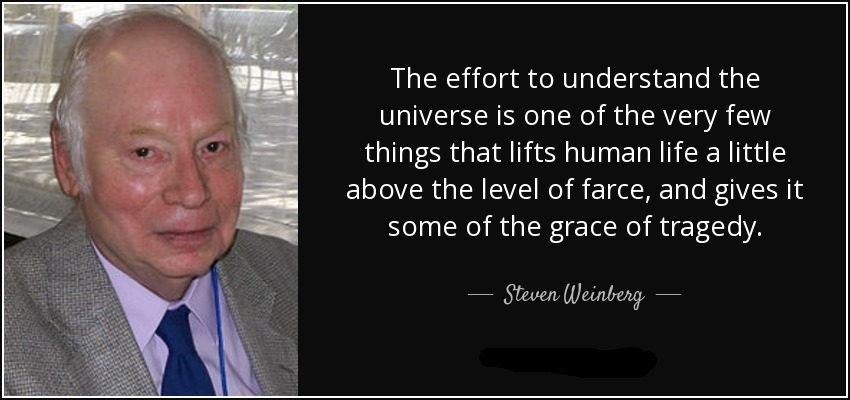Steven Weinberg died today.

For all the talk of unification in physics, there are few examples. Newton unified terrestrial and celestial gravity — apples and planets. Maxwell unified electricity and magnetism. Weinberg, Glashow and Salam unified electromagnetism and the weak force.

This unification is curious, since it starts with two other kinds of force and uses another particle, now called the Higgs boson, to split these force in a different way, into the forces we now see... while giving particles mass!

While curious, the Glashow–Salam–Weinberg theory predicted the W, Z and Higgs bosons with the properties we now see — along with all other details of the electromagnetic and weak forces. So, it is a magnificent success, opening the door to deeper mysteries.

For beginners, I recommend Weinberg's book The First Three Minutes. For those who know particle physics, I recommend his Nobel lecture:

It's striking how little more we know for sure about fundamental physics now than we did then!

July 26, 2021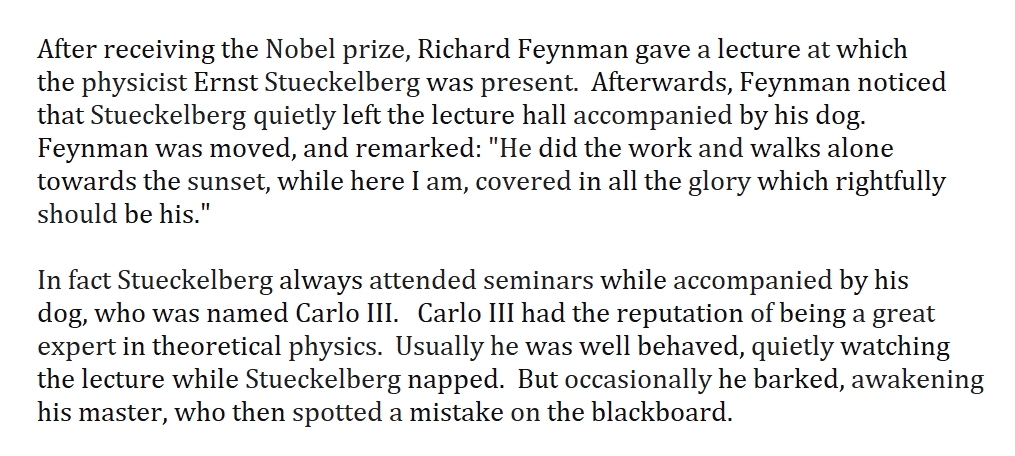Some physicists win Nobels, while others just have great dogs.

Who was Stueckelberg, and why did Feynman think he deserved a Nobel? What did he do that was so great?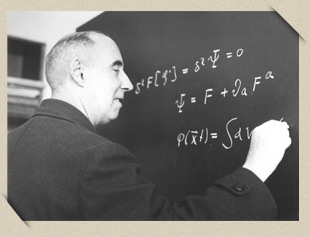His full name was Baron Ernst Carl Gerlach Stueckelberg von Breidenbach zu Breidenstein und Melsbach. Born in 1905, he was a master of quantum field theory, the first to do many things... but he published in minor journals, so few recognized his greatness until the 1990s.

Here are some things he did:

• In 1934 he devised a fully Lorentz-invariant perturbation theory for quantum fields. (A big deal.)
• In 1935 he developed a theory of mesons carrying the nuclear force. (Yukawa won the Nobel for this in 1949.)
• In 1938 he came up with a primitive version of the Higgs mechanism. He also discovered conservation of baryon number - an important conservation law.
• In 1941 he proposed that positrons are electrons traveling backward in time... an idea Feynman also had, later.
• In 1943 he came up with a renormalization program to tackle the problems of infinities in quantum field theory, but his paper was rejected by the Physical Review.
• In 1953 he and André Petermann discovered the renormalization group... before others did.
His work was not completely unrecognized: in 1976 he got the Max Planck medal. But physics would have advanced faster if more people had paid attention to him!

It's a good lesson: don't just pay attention to the bigshots in your field. Look for good ideas.